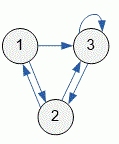Competitions

# Count number of edges

The directed graph is given with an adjacency matrix. Find the number of edges in a graph.

#### Input

The first line contains the number of vertices in a graph n (1n100). Then given n lines with n numbers 0 or 1 - the adjacency matrix of the graph.

#### Output

Print the number of edges in a graph.Time limit 1 second
Memory limit 128 MiB
Input example #1
3
0 1 1
1 0 1
0 1 1

Output example #1
6